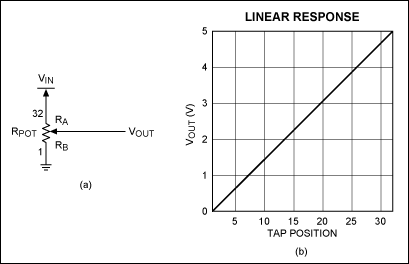# Use a Linear Potentiometer to Create a Nonlinear Transfer Function for Audio Volume Control

### Abstract

Linear digital potentiometers (pots) can be used in nonlinear applications, such as adjusting volume control in audio applications. This article discusses how to create a \"pseudologarithmic\" taper digital potentiometer (pot). This is achieved by simply adding a single resistor to the end of the digital pot when configured as a variable resistor.

### Introduction

To meet specific dynamic-range requirements, many applications require circuits that have a nonlinear transfer function. From linearizing a circuit element's response to providing a logarithmic output for volume control, this function is useful in a variety of engineering applications. Linear-taper digital potentiometers (pots) can provide nonlinear transfer functions in simple voltage-divider configurations. For the following examples, the 50kΩ version of the MAX5160 digital pot is used to describe these types of transfer functions.

### Generating a Linear Transfer Function

Typical voltage-divider configurations are shown in Figures 1a, 2a, and 3a. For each of these circuits, assume that VIN represents a low-impedance voltage source, and that VOUT represents a high-impedance input node. Figure 1a depicts a simple voltage-divider with a transfer function of VOUT = VIN(RB/RPOT), where RPOT = RA + RB. RA and RB represent the resistances from VIN to the wiper, and from the wiper to ground, respectively. Stepping the wiper from the bottom of the pot to the top, with VIN at a constant 5V level, generates a linear transfer function. This is shown in the linear-response plot in Figure 1b. A link to an Excel® spreadsheet is provided at the end of this article, to help you calculate VOUT versus tap-position data.Adding a series resistor to the circuit (R1 in Figure 2a) yields an entirely different result. Now this circuit's transfer function more closely resembles a logarithmic curve and is characterized by the equation VOUT = VIN(RB/(R1 + RB)). By varying the value of R1, which in this case is 10kΩ, the "pseudologarithmic" transfer function can be customized to meet the actual needs of the circuit. A graph of the transfer function is shown in Figure 2b. One possible drawback to this circuit, as compared to a log-taper digital pot, is that VOUT can only approach the full-scale value of VIN. VOUT cannot reach VIN. Therefore, there is some loss of the output's dynamic range; the magnitude of that loss depends on the value chosen for R1. Using a log-taper digital pot, however, limits the response to that particular pot's specifications (1dB/step, 2dB/step, etc). There are trade-offs for both topologies, depending on the specifications needed.Lastly, swapping the positions of R1 and the MAX5160 yields the circuit shown in Figure 3a. This configuration alters the response of the output to form more of an inverse-logarithmic function. The transfer function for this circuit is given as VOUT = VIN(R1/(R1 + RB)) and is shown in Figure 3b. As in the previous example, the value chosen for R1 affects the dynamic range of the output. This circuit's output does not extend to the ground rail.For simple applications, such as the examples given, the linked spreadsheet can be manipulated to cover a wide range of digital pots and circuit values. The equations in the spreadsheet do not include the MAX5160's ±25% end-to-end resistance tolerance, but they can be easily adapted to include it, as well as other parameters and pots. Follow the steps outlined below:

1. After opening the spreadsheet, select the tab at the bottom that corresponds to the type of nonlinear response desired (linear, log response, or inverse-log response). Enter the value of VIN in cell A2.
2. Enter the value of R1 in cell B2.
3. Enter the value of the pot resistance in cell C2.
4. Enter the total number of steps provided by the pot in cell D2.
5. Copy the values found in row 33 of columns E, F, G, and H, and paste them in each subsequent row until the number of steps in column E equals the total number of steps provided by the pot (and entered in cell D2). In other words, for a 64-step pot, copy the E33, F33, G33, and H33 values and paste them in all subsequent rows through row 65.
6. For older versions of Excel, follow the steps outlined in 6a. For newer versions of Excel, follow the steps outlined in 6b.

• Click on the graph in the spreadsheet, and the word "Chart" will appear in the menu above the toolbar at the top of the screen. If a separate Chart Toolbar pops up on the screen, close it. Click on "Chart" at the top of the screen. From the pulldown menu, click on "Source Data." A separate window will appear with the data range of cells highlighted. Now click and drag the pointer down column H (VOUT) of the spreadsheet until the cells of interest are outlined. Then click OK in the Source Data window. The graph now depicts the new VOUT versus tap-position data.
• For newer versions of Excel, right-click on the graph and choose Select Data from the pulldown menu that appears. A Select Data Source window will open and a data range of cells will become selected. Click and drag a box over the cells in column H (VOUT), until the cells of interest are selected. The formula in the Select Data Source window will change automatically as you do this. Once you have the correct cells selected, click the OK button in the window. The graph now depicts the new VOUT versus tap-position data.

The examples given are generic in their scope and have not included several parameters, such as wiper resistance, zero- and full-scale error, end-to-end resistance tolerance, and temperature coefficients. However, the spreadsheet can be modified to include these specifications.